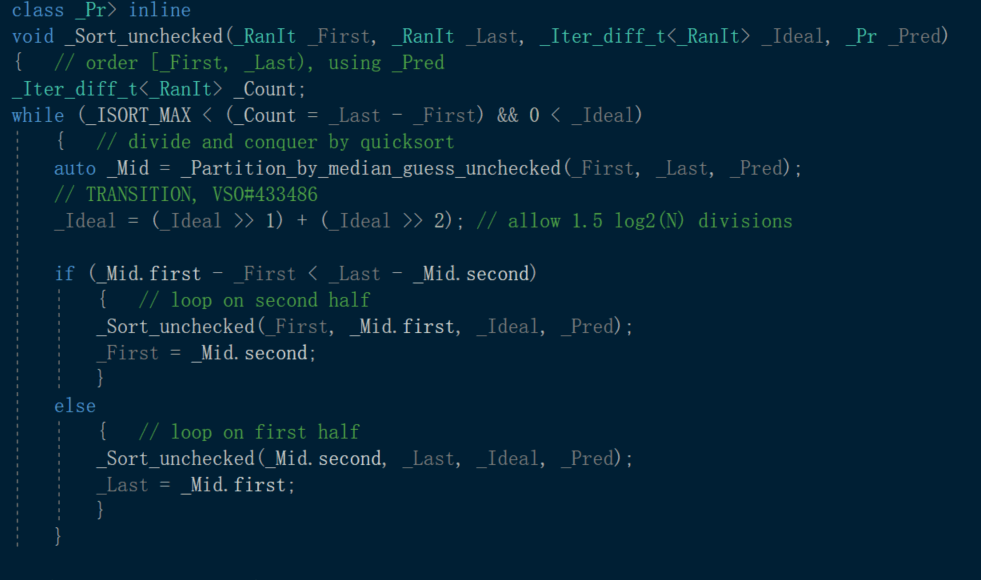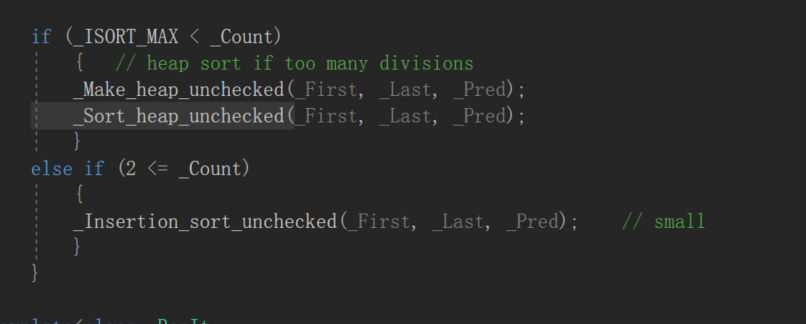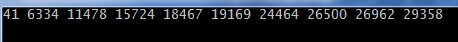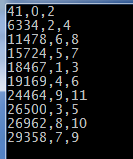• 在OJ上遇到一道题，大概是统计各类数据的个数，然后排序。题目描述如下： 如果统计的个数相同，则按照ASII码由小到大排序输出 。如果有其他字符，则对这些字符不用进行统计。实现以下接口： 输入一个字符串，对...
      在OJ上遇到一道题，大概是统计各类数据的个数，然后排序。题目描述如下：

如果统计的个数相同，则按照ASII码由小到大排序输出 。如果有其他字符，则对这些字符不用进行统计。实现以下接口：    输入一个字符串，对字符中的各个英文字符，数字，空格进行统计（可反复调用）    按照统计个数由多到少输出统计结果，如果统计的个数相同，则按照ASII码由小到大排序输出    清空目前的统计结果，重新统计调用者会保证：输入的字符串以‘\0’结尾。
//统计字符
#include <vector>
#include<iostream>
#include<string>
#include<cctype>
#include <algorithm>
using namespace std;

int main()
{
string str;
vector<int> v;
vector<int>::iterator iter = v.begin();
getline(cin,str);
int len = str.size(), letter_n = 0, digit_n = 0, space_n = 0;
for (int i = 0; i<len; i++)
{
if (isalpha(str[i]))
letter_n++;
else if (isdigit(str[i]))
digit_n++;
else if (isspace(str[i]))
space_n++;
}
v.push_back(letter_n);
v.push_back(digit_n);
v.push_back(space_n);
/* for (iter = v.begin(); iter != v.end(); iter++)
cout << *iter<<',';
cout << endl; */ //普通迭代器输出
cout << "反向迭代器输出: " << endl;
vector<int>::reverse_iterator it = v.rbegin();
while (it != v.rend())
{
cout << *it << ",";
++it;
}
return 0;
}

展开全文• 3、vector的sort排序 4、翻转vector中的所有元素 5、find()函数的用法 6、vector实战（这里写的是我在最开始用vector时候犯的错误） 1、基本用法： 头文件： #include<vector> 定义vector： 整数型...
终于把自己的个人博客安排上啦！

欢迎访问我的个人博客 XJHui's Blog。

vector用法目录：

1、基本用法

2、vector的删除操作

3、vector的sort排序

4、翻转vector中的所有元素

5、find()函数的用法

6、vector实战（这里写的是我在最开始用vector时候犯的错误）

1、基本用法：

头文件：

#include<vector>

定义vector：

整数型：    vector<int>a;
字符型：    vector<char>a;
字符串型：  vector<string>a; (注意字符串型输入输出用cin和cout)

固定vector的大小：
vector<int>a(105);


在容器最后存入新元素：

for(int i=0;i<10;i++){
b.push_back(i);
}

将字符数组中的数据赋值给容器：

1、遍历赋值：
char a[MAX+5];
vector<char>vc;
cin>>a;
int la=strlen(a);
for(int i=0;i<la;i++)
vc.push_back(a[i]);
2、定义容器时赋值：
char a[MAX+5];
cin>>a;
int la=strlen(a);
vector<char>vc(a,a+la);

判断容器的长度：

printf("%d\n",b.size());

遍历容器中元素：

1、遍历输出
for(int i=0;i<vc.size();i++)
printf("%c",vc[i]);
printf("\n");
2、错误示例：
for(int i=vc.begin();i<vc.end();i++){
cout<<vc[i]<<" ";
}
cout<<endl;

清空容器、判断是否为空、删除最后元素：

清空容器： a.clear();//清空a中的元素
判断容器是否空：a.empty();//判断a是否为空，空则返回ture,不空则返回false
删除容器最后的元素：  a.pop_back(); ///括号内不可以添加index删除指定元素

2、vector的删除操作

vector很好的一点就是可以实现删除操作，用它来做模拟很好实现。

1、函数使用：
vc.erase(vc.begin(),vc.end()); ///vc.erase()可以删除一个或多个元素
2、说明：
vc.erase()函数中添加的地址范围是左闭右开区间,删除内容后，如果后面还有元素，会自动补全空位。
3、注意：
///vc.erase()函数中不是直接加要删除的内容，下面例子是错误的。
vc.erase('a');          

3、vector的sort排序（加#include<algorithm>头文件）

将元素从小到大的顺序排列（默认）：

int c1[]={1,3,11,2,66,22,-10};
vector<int>c(c1,c1+7); ///赋初值
sort(c.begin(),c.end());
for(int i=0;i<c.size();i++){
printf("%d ",c[i]);
}
printf("\n");

重写cmp函数按要求排序：

#include<stdio.h>
#include<vector>
#include<algorithm>
using namespace std;
bool cmp(int x,int y) ///cmp函数传参的类型不是vector<int>型，是vector中元素类型,即int型
{
return x>y;
}
int main()
{
int c1[]={1,3,11,2,66,22,-10};
vector<int> c(c1,c1+7);
sort(c.begin(),c.end(),cmp);
for(int i=0;i<c.size();i++){
printf("%d ",c[i]);
}
printf("\n");
return 0;
}


vector<node>(结构体)的cmp函数与其类似

4、翻转vector中的所有元素：

int d1[]={1,3,11,2,66,22,-10};
vector<int> d(d1,d1+7);

reverse(d.begin(),d.end()); ///不是d.reverse()

for(int i=0;i<d.size();i++){
printf("%d ",d[i]);
}
printf("\n");

5、find()函数的用法：

int temp1[]={ 1,3,2,4,5,0 };
vector<int> temp(temp1,temp1+6);

vector<int>::iterator it=find(temp.begin(),temp.end(),3);///返回的是地址

int index=&*it-&temp;///放入迭代器中得到容器中的位置

printf("%d\n",index);

6、vector实战：（这里写的是我在最开始用vector时候犯的错误）

vector没申请不能直接赋值

    vector<int>a;
for(int i=0;i<10;i++){
a[i]=5; ///vector[i]根本没被申请，所以不可以直接赋值
}

    vector<int>a;
for(int i=0;i<10;i++){
a.push_back(i); ///申请a后，下面可以直接改变它的值
}
a=6;

    vector<int>a(10);///先申请一个大小为10的向量后也可以直接改变a的值
a=6; 

（上面只是vector的一部分内容，后续会逐渐补充，不足请评论）

如果对博客搭建感兴趣可以看我另一篇博客：Hexo博客框架攻略

也欢迎到我的个人博客评论、留言：XJHui's Blog

展开全文• 最近写c++的时候发现vector自带排序算法：sort，并且这玩意可以自定义排序的规则。 索性去内部看了一下 比较有意思的就是内部先用的折半排序的思想，然后如果数目太多会自动替换成下图的堆排序。 看起来排序...
最近写c++的时候发现vector自带排序算法：sort，并且这玩意可以自定义排序的规则。

索性去内部看了一下比较有意思的就是内部先用的折半排序的思想，然后如果数目太多会自动替换成下图的堆排序。看起来排序效率会比自己写的好。

展开全文• 参考资料：关于C++中vector和set使用sort方法进行排序 作者注：这篇文章写得相当全面，包括对...sort函数默认从小到大的排序。 一般类型的排序（int，double，char） vector<int> vec; for (int i = 0;...
参考资料：关于C++中vector和set使用sort方法进行排序
作者注：这篇文章写得相当全面，包括对vector和set中不同数据类型（包括结构体）的排序，还有一些还没看懂……特作此摘录，供当前及日后的学习

sort函数默认从小到大的排序。

一般类型的排序（int，double，char）

    vector<int> vec;
for (int i = 0; i < 10;i++)
{
vec.push_back(rand());
}
sort(vec.begin(), vec.end());
for (vector<int>::iterator it = vec.begin(); it < vec.end(); it++)
{
cout << *it << " ";//注意这里使用*表示解引用
}

1
2
3
4
5
6
7
8
9
10
结果：结构体类型的排序（struct）

结构体定义如下：（试验样本）

typedef struct ClassDis
{
double distance;
int class1;
int class2;
}ClassDis;

1
2
3
4
5
6
需要自己定义一个比较函数（因为sort默认从小到大排序，因此我们只需要定义什么情况属于“小于”就行了）

//自定义“小于”
bool comp(const ClassDis &a, const ClassDis &b)
{
return a.distance < b.distance;
}

1
2
3
4
5
    vector<ClassDis> ddd;
//随机赋值
for (double a = 0; a < 10;a++)
{
ClassDis aaa{ (double)rand(), (int)a, (int)(a + 2) };
ddd.push_back(aaa);
}
//sort
sort(ddd.begin(), ddd.end(), comp);
//输出结果
for (vector<ClassDis>::iterator it = ddd.begin(); it < ddd.end(); it++)
{
cout << it->distance << "," << it->class1 << "," << it->class2 << endl;
}

1
2
3
4
5
6
7
8
9
10
11
12
13
14
结果：展开全文• 绝对值排序 Problem Description 输入n(n<=100)个整数，按照绝对值从大到小排序后输出。题目保证对于每一个测试实例，所有的数的绝对值都不相等。 Input 输入数据有多组，每组占一行，每行的第一个数字为n,接着是...算法
• C++中当 vector 中的数据类型为基本类型时我们调用std::sort函数很容易实现 vector中数据成员的升序和降序排序，然而当vector中的数据类型为自定义结构体类型时，我们该怎样实现升序与降序排列呢？有两种方法，下面...
• ## C++ vector排序

万次阅读 2018-08-08 14:37:17
使用sort（）函数（要添加引用algorithm）可以很方便地对数组进行排序，搭配自定义的compare函数可以实现多种对数据进行排序的方式，同样，对vector排序也可以使用sort函数，一般来说，在对vector进行排序地时候，要...C++
• ## vector排序

千次阅读 2012-08-31 09:45:45
C++中当 vector 中的数据类型为基本类型时我们调用std::sort函数很容易实现 vector中数据成员的升序和降序排序，然而当vector中的数据类型为自定义结构体类型时，我们该怎样实现升序与降序排列呢？有两种方法，iterator 算法 struct google
• 向量容器vector 动态数组，可以在运行阶段设置长度 具有数组的快速索引方式 可以插入和删除元素 定义和初始化 vector vec1; 1.创建vector数组: #include <iostream> #include <vector> using ...c++ 数据结构
• 的解决方法，他将名字和记录一起存储在vector中，然后用sort函数vector排序，排序规则为优先按照字典顺序排列名字，如果名字相同则按照时间顺序（通过对时、分、秒加和来衡量）排列，这样排列完毕后的记录是按照...算法 PAT
• 一、排序函数sort()的排序（涉及到vector容器） #include <string.h> #include <vector> #include <iostream> #include <algorithm> using namespace std; int main() { vector<int&...c++ 算法
• #include <string> #include <iostream> #include <vector> #include <... //sort排序必备头文件 using namespace std; int main() { vector<int>c;​​​​​​​​​​​​...
• C++中当 vector 中的数据类型为基本类型时我们调用std::sort函数很容易实现 vector中数据成员的升序和降序排序，然而当vector中的数据类型为自定义结构体类型时，我们该怎样实现升序与降序排列呢？ 方法1：重载...
• ## sort()函数排序vector

千次阅读 2019-05-05 14:33:26
sort()函数默认的排序方式为升序，如果需要降序排序，可以自定义排序函数 bool comp(int x ,int y) { return x > y; } 对vector进行排序时 sort(vec.begin(),vec.end(),comp); 这样排序出的vector就是降序...
• C++中当 vector 中的数据类型为基本类型时我们调用std::sort函数很容易实现 vector中数据成员的升序和降序排序，然而当vector中的数据类型为自定义结构体类型时，我们该怎样实现升序与降序排列呢？有两种方法，下面...
• ## C++ vector常用函数

千次阅读 2018-03-11 21:25:19
一、vector排序。#include&lt;bits/stdc++.h&gt; using namespace std; int main() { vector&lt;int&gt;p; for(int i=10;i&gt;=0;i--) p.push_back(i); for(int i=0;i&lt;p.size();i++)...
• algorithm库的sort() 排序函数的使用方法 参考：C++ STL sort 函数的用法 1、sort函数包含在头文件为#include的c++标准库中 2、sort函数有三个参数： （1）第一个是要排序的数组的起始地址。 （2）第二个是结束的...c++
• 主要是用了std::sort 函数 这里只写一种lambda写法。 std::vector<std::map<std::string, std::string>> tempArr; std::sort(tempArr.begin(), tempArr.end(), [](const map<string, string> &...
• vector是线性容器,它的元素严格的按照线性序列排序,和动态数组很相似,和数组一样,它的元素存储在一块连续的存储空间中,这也意味着我们不仅可以使用迭代器(iterator)访问元素,还可以使用指针的偏移方式访问,和常规......